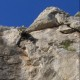# Pixel position reconstruction

Sometimes we need to know, in a fragment program, a pixel’s position in eye space. We usually need this in postprocessing operations, where we will tipically send an eye-facing quad filling the whole screen, executing an specific fragment program along with information about the scene (usually textures that contain surface normals, depth, materials, etc) that has been previously rendered in a previous pass.

So, during this postprocessing step, each fragment belonging to this quad corresponds to a little area on the surface of the previously rendered scene, although by now we only know its position within the eye-facing quad being rendered.

The question is: Which was its exact position in the scene in eye space?

## First rendering pass

In this pass, the original scene’s geometry is rendered in a FBO, and the depth of each fragment is stored into an output texture (attached to the frame buffer object). In the following example, we can see how this depth is stored in the output texture’s red channel:

 12 // Storing Z in non linear range [0,1] gl_FragColor.r = gl_FragCoord.z;

It is important that the output texture is configured properly so it has at least a precission of 16 bits per color channel, as 8 bits per channel are not enough to compute most postprocessing effects involving depth properly.

## Second rendering pass

Here, an eye-facing quad filling the whole screen is rendered. Its texture coordinates must be properly defined in its vertices. The previous rendering pass’ output texture is used now as input (inputTexture). Also, we are going to need the values defining the vision frustum: znear, zfar, left, right, bottom and top.

 123456789101112 // Input texture uniform sampler2D inputTexture; // Frustum definition values uniform float n; // znear uniform float f; // zfar uniform float l; // left uniform float r; // right uniform float b; // bottom uniform float t; // top // Quad/texture size in pixels uniform float textureWidth; uniform float textureHeight;

### Z reconstruction

We can calculate the Z coordinate in eye space by taking the depth value rendered in the first rendering pass, converting it into NDC (normalized device coordinates) and applying the following formula:

 12345678 // z in non linear range [0,1] float zpixel = inputTexture.r; // conversion into NDC [-1,1] float zndc = zpixel * 2.0 - 1.0; // conversion into eye space float zeye = 2*f*n / (zndc*(f-n)-(f+n));

### X and Y reconstruction

First of all we need to know X and Y coordinates in NDC space:

 123 // Converting from pixel coordinates to NDC float xndc = gl_FragCoord.x/textureWidth * 2.0 - 1.0; float yndc = gl_FragCoord.y/textureHeight * 2.0 - 1.0;

Once we have all this stuff, we can compute X and Y in eye space by using the following formulae (we are simply unprojecting X and Y from NDC to eye space):

 12 float xeye = -zeye*(xndc*(r-l)+(r+l))/(2.0*n); float yeye = -zeye*(yndc*(t-b)+(t+b))/(2.0*n);

And finally:

 1 vec3 eyecoords = vec3(xeye, yeye, zeye);
1.Utsav Pardasani says:

Why do you need the first pass? Couldn’t you just do

float zpixel = gl_FragCoord.z;

?

2.Utsav Pardasani says:

Nevermind – I understand now. Since you are rendering to a quad in the 2nd pass – you won’t have access to that depth.

Cool.

3.txutxi says:

You are right and the first pass (and the whole explanation above) is not necessary at all if you are performing forward rendering. But I was focusing post-processing operations (e.g. deferred shading, ssao, etc).

These techniques tipically throw the original geometry in the first pass and store that pass’ output into some 2d textures (here is where gl_FragCoord.z is stored; other components like color, normal vectors and so on can also be stored depending on our needs).
The following post-processing render passes don’t know the original geometry, we throw screen-filling quads (whose gl_FragCoord.z corresponds to the quad’s depth) with a shader that uses the first pass output textures, and these textures contain all information we need for the final image composition (the original geometry depth in above mentioned case, as we need it for the fragment position reconstruction).

All the original geometry coordinages (x,y,z) could also have been stored into these output textures and used as input textures in the following post-processing steps, thus avoiding all the pixel position reconstruction stuff… this was simply a way to efficiently pass this information without wasting more resourcesI don’t remember right now but think that the resource I looked at when I was developing this is here

4.txutxi says:

Indeed5.ataxxdog says:

Thanks for the helpful post! For reconstructing depth, it is not necessary to have the n and f uniforms, as long as the projection matrix in the second pass is the same as the first. You can simply do:

float zeye = gl_ProjectionMatrix / (zndc – gl_ProjectionMatrix);

6.txutxi says:

Thanks for your reply! At least one could go with the ‘n’ and ‘f’ uniforms approach whenever gl_ProjectionMatrix is not set in the second step7.snailman153624 says:

If you were to use gl_ProjectionMatrix, I think the correct equation is:

float zeye = gl_ProjectionMatrix/(-2.0* zndc);

Note that at least for AMD graphics cards, the GPU Shader Analyzer tool doesn’t show any performance difference vs. using uniforms (although this is simpler and requires less input from the calling application software).

8.snailman153624 says:

Correction:

float zeye = gl_ProjectionMatrix/(zndc – 1.0);

9.snailman153624 says:

Ha, basic algebra keeps kicking my butt.

float zeye = -gl_ProjectionMatrix/(zndc + gl_ProjectionMatrix);

My previous equation seemed to work because it discarded a -2n in the denominator, which is largely inconsequential (but not 100% accurate).

However, I contend ataxxdog’s equation is incorrect.